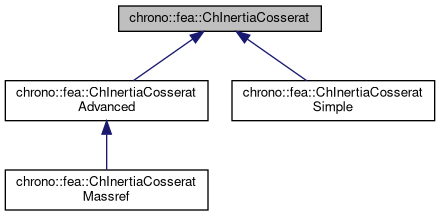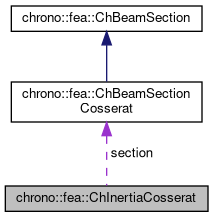chrono::fea::ChInertiaCosserat Class Referenceabstract

## Description

Base class for ineri tal properties (mass, moment of inertia) of beam sections of Cosserat type.

This can be shared between multiple beams.

#include <ChBeamSectionCosserat.h>

Inheritance diagram for chrono::fea::ChInertiaCosserat:[legend]
Collaboration diagram for chrono::fea::ChInertiaCosserat:[legend]

## Public Member Functions

virtual void ComputeInertiaMatrix (ChMatrixNM< double, 6, 6 > &M)=0
Compute the 6x6 sectional inertia matrix, as in {x_momentum,w_momentum}=[Mm]{xvel,wvel} The matrix is computed in the material reference (i.e. More...

virtual void ComputeQuadraticTerms (ChVector<> &mF, ChVector<> &mT, const ChVector<> &mW)=0
Compute the values of inertial force & torque depending on quadratic velocity terms, that is the gyroscopic torque and the centrifugal term (if any). More...

virtual double GetMassPerUnitLength ()=0
Compute mass per unit length, ex.SI units [kg/m]. More...

## Public Attributes

ChBeamSectionCosseratsection

## ◆ ComputeInertiaMatrix()

 virtual void chrono::fea::ChInertiaCosserat::ComputeInertiaMatrix ( ChMatrixNM< double, 6, 6 > & M )
pure virtual

Compute the 6x6 sectional inertia matrix, as in {x_momentum,w_momentum}=[Mm]{xvel,wvel} The matrix is computed in the material reference (i.e.

it is the sectional mass matrix)

Parameters
 M 6x6 sectional mass matrix values here

 virtual void chrono::fea::ChInertiaCosserat::ComputeQuadraticTerms ( ChVector<> & mF, ChVector<> & mT, const ChVector<> & mW )
pure virtual

Compute the values of inertial force & torque depending on quadratic velocity terms, that is the gyroscopic torque and the centrifugal term (if any).

All terms expressed in the material reference, ie. the reference in the centerline of the section.

Parameters
 mF centrifugal term (if any) returned here mT gyroscopic term returned here mW current angular velocity of section, in material frame

## ◆ GetMassPerUnitLength()

 virtual double chrono::fea::ChInertiaCosserat::GetMassPerUnitLength ( )
pure virtual

Compute mass per unit length, ex.SI units [kg/m].

This is also the(0, 0) element in the sectional inertia matrix.